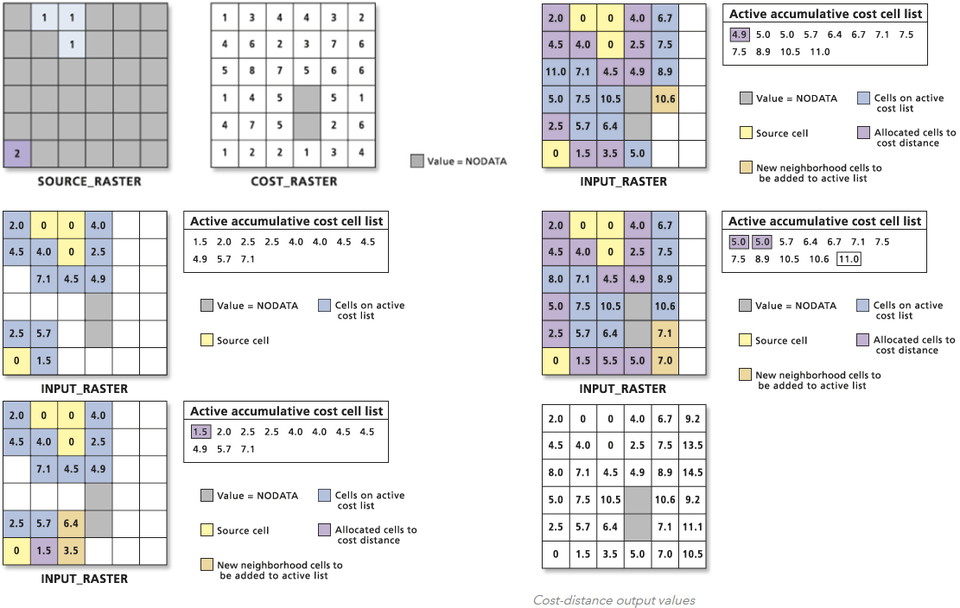$\newcommand\si{\mathrm{#1}} \newcommand\SI{#1\,\si{#2}} \newcommand\matr{\mathbf{#1}} \DeclareMathOperator*{\argmax}{arg\,max} \DeclareMathOperator*{\argmin}{arg\,min}$

# GIS process models

Institute for Environmental and Spatial Analysis...University of North Georgia

## 1   Process models

Simulate real-world processes.

A prior models model processes for which a body of theory has yet to be established (e.g., impacts of global warming).

A posterior models are designed to explore established theories (e.g., avalanche-prone area).

## 2   Classification

Natural analogue models use actual events or real objects to construct a model (different place or time).

Scale analogue models are scaled down and generalized replicas of reality (e.g., aerial photos, topo maps).

Conceptual process models are often expressed in verbal or graphical form to describe interactions between features (e.g., system diagrams).

## 3   Mathematical process models

Deterministic model: Only one possible answer for given inputs (linear relationship)

Stochastic model: Considers uncertainty and a range of possible outcomes based on inputs, and assign probabilities (many variables)

Optimization model: Constructed to minimize or maximize some aspects of the model’s output

## 4   Avalanche prediction

### 4.1   Analogue model of avalanche prediction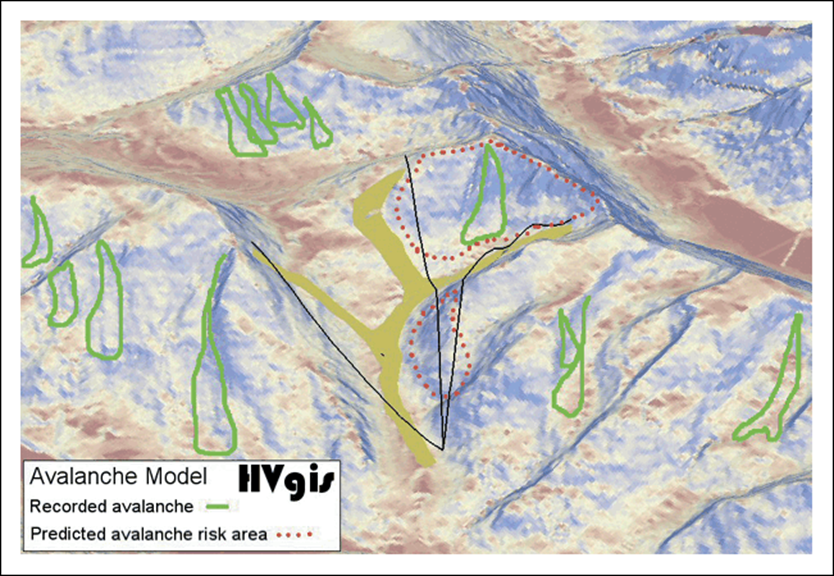### 4.2   Conceptual model of avalanche prediction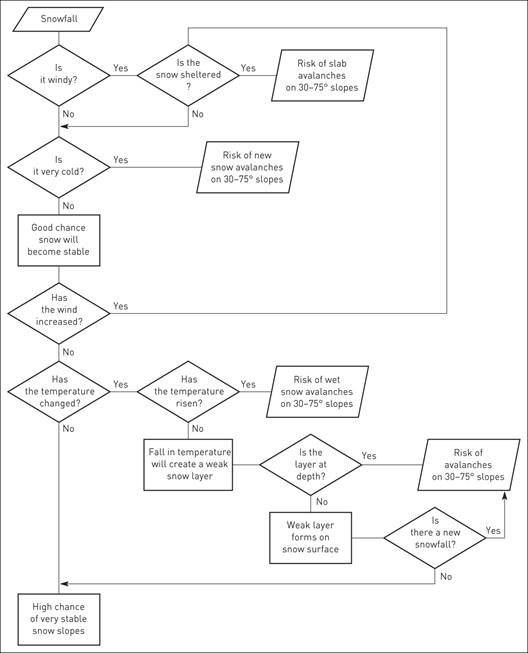### 4.3   Slope and avalanche size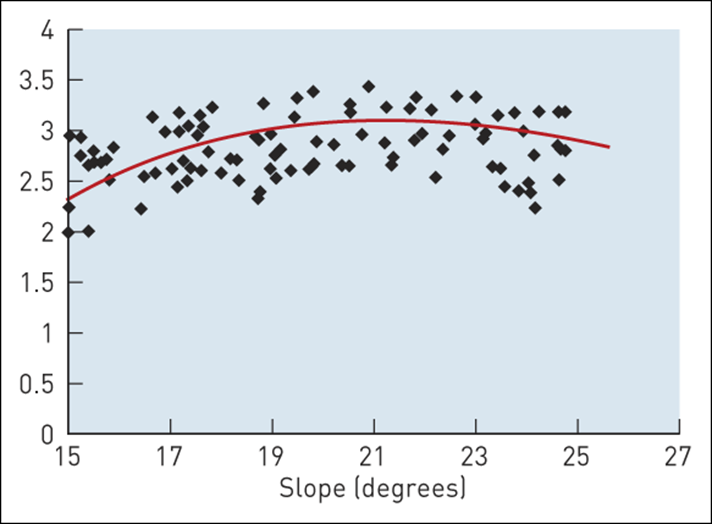## 5   Process models

Dynamic: Changes over time

## 6   Estimating emissions

### 6.1   GIS framework for estimating emissions### 6.2   Estimates of NOx emissions from all sources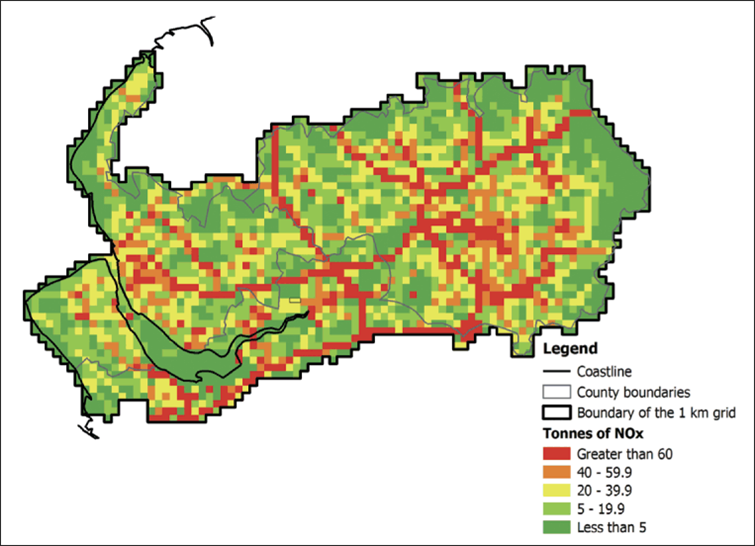### 6.3   Estimates of NOx emissions from motorways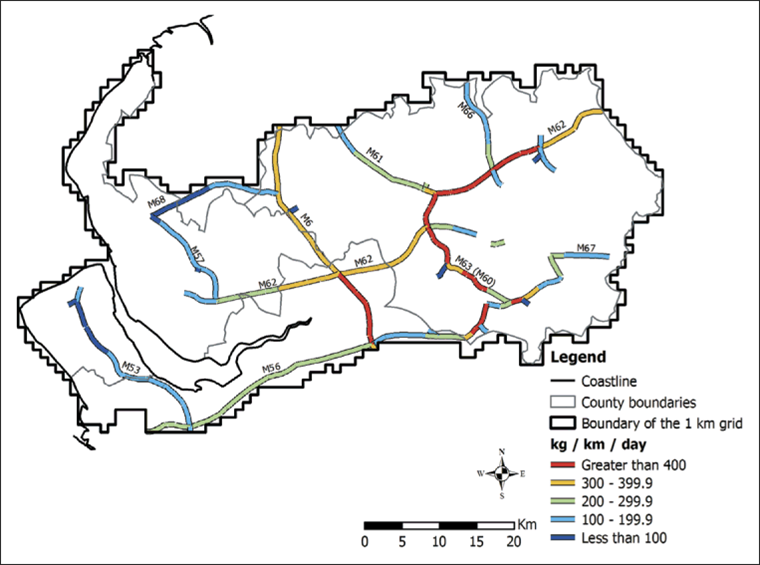## 7   Conceptual model of forest fires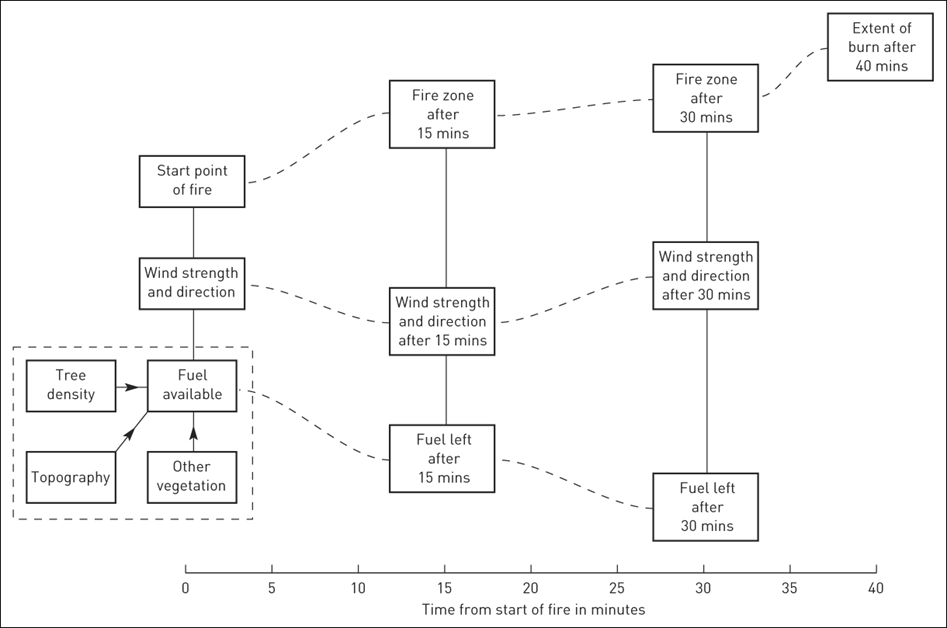## 8   Multi-criteria evaluation (MCE)

Map overlays have problems:

• Difficult to comprehend when many layers are involved
• Most overlay procedures in GIS assume equal importance of layers
• Threshold values are important

### 8.1   What is MCE?

MCE is the application of GIS to support the process of decision making by evaluating multiple criteria at the same time.

MCE is a common method to aggregate and analyze a variety of criteria to facilitate decision making and problem solving.

Each criterion is represented as a layer of geographic data (standardized suitability mapping) and assigned a weight.

Who decides weights? Public input

### 8.2   Linear weighted summation### 8.3   Weighting data layers for house hunting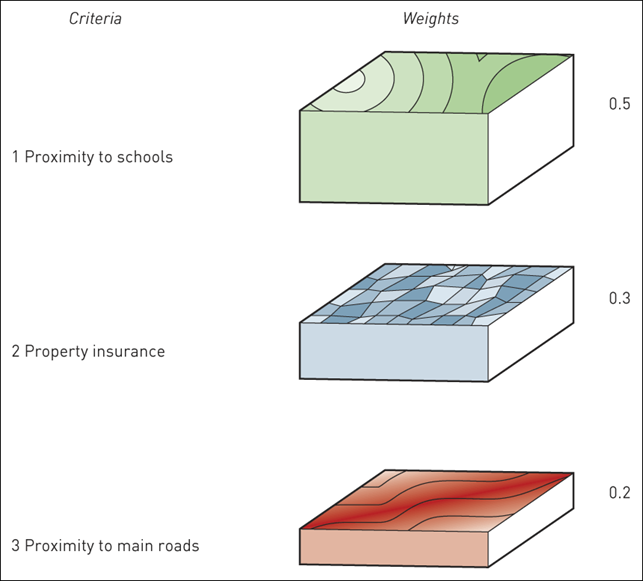## 9   Cost distance

Used to create the least-cost path between a source and a destination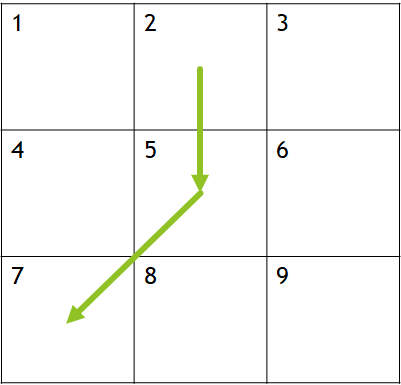### 9.1   Cost distance calculation